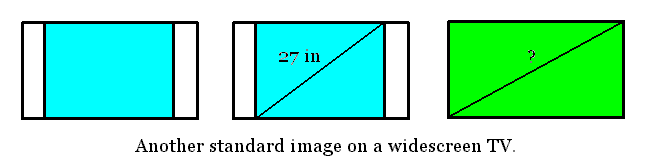THE PYTHAGOREAN THEOREM
(Covers "Standard of Learing" items 5.14, 5.15, 6.15, 8.10)

Vocabulary and "Facts"

1. The Parallel Postulate says that "Through a given point not on a given line can be drawn only one line parallel to the given line" (see problem 30 of section 4.6 of The Heart of Mathematics).
2. From the Parallel Postulate it follows that: "The sum of the measures of the angles of a triangle is 180o."
3. A right angle is a 90o angle (or half of a straight angle). A triangle containing a right angle is a right triangle. The hypotenuse of a right triangle is the side opposite the right angle.
4. From the Parallel Postulate follows: The Pythagorean Theorem. In a right triangle, the square of the length of the hypotenuse is equal to the sum of the squares of the lengths of the other two sides. This means that if the hypotenuse has length c and the other two "legs" are lengths a and b, then a2 + b2 = c2.
5. Angles with measures between 0o and 90o (excluding 0o and 90o) are acute angles (The Heart of Mathematics jokingly calls these "wrong" angles). Two acute angles whose measures add up to 90o are complementary (The Heart of Mathematics says for this "... two wrongs actually do make a right").

Pythagoras and Manipulatives
(SOL: K11-K13, 1.16, 1.17, 4.17)
1. Problem 5 from section 4.1 gives a picture of how the Pythagorean Theorem can be thought of in terms of areas (this is how the Greeks would have conceived it):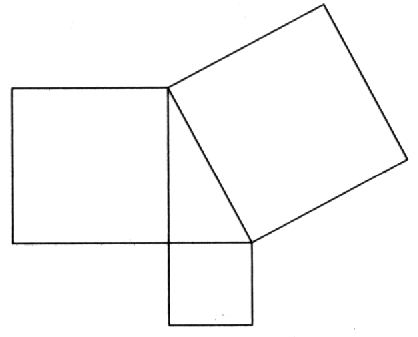2. The "Manipulatives Kit" includes the "Pythagorean Puzzle Proof." It can be used to show that a square of area c2 can be rearranged to form two squares, one with area a2 and one with area b2. To complete the "proof," we must do problem 17 from this section.
3. The following two pictures are the same. Shade in an area of size a2 + b2 in the left picture and an area of size c2 in the right. How does this relate to the Pythagorean Theorem and where is the right triangle?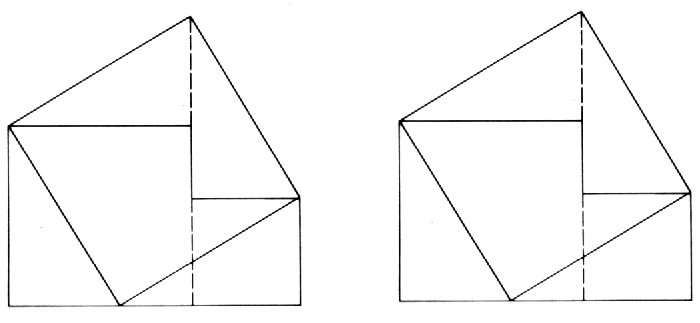This construction was known to Tabit ibn Qorra (828-901) and rediscovered by H. Perigal in 1873 (from An Introduction to the History of Mathematics, Howard Eves, Saunders College Publishing, 1983).
4. Another demonstration of the Pythagorean Theorem involves the following pictures and was presented in 1917 by H.E. Dudeny (from Eves). The brackets indicate equal distances.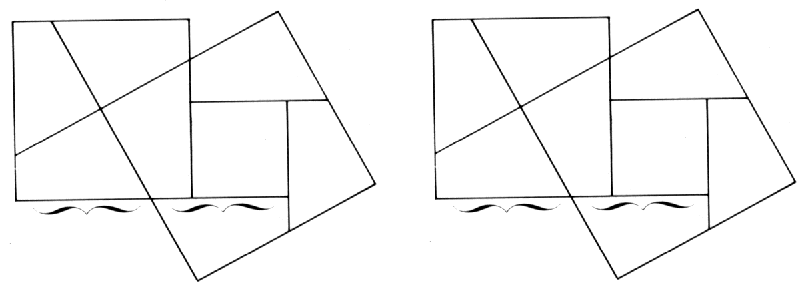5. Pieces can be added to the following picture to demonstrate the Pythagorean Theorem (from Eves). This one is rumored to be due to Leonardo da Vinci (1452-1519).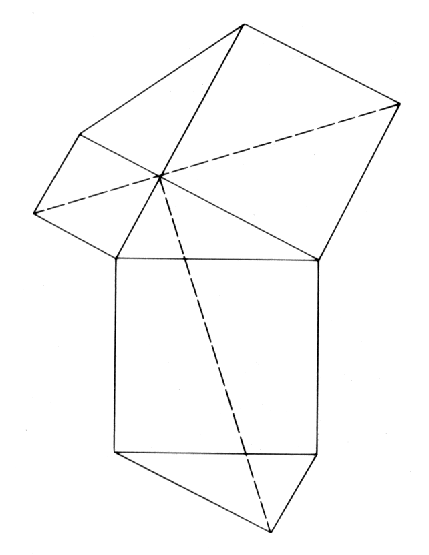6. Use multiplication and areas to explain how the following relates to the Pythagorean Theorem (from Eves).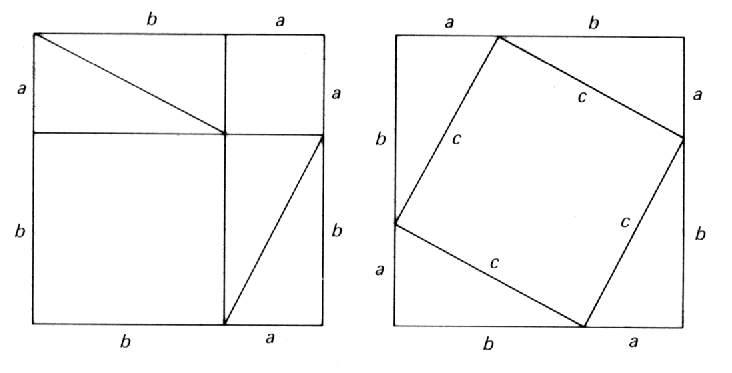Euclid's original proof is based on this picture (from Eves). Any ideas how the proof goes?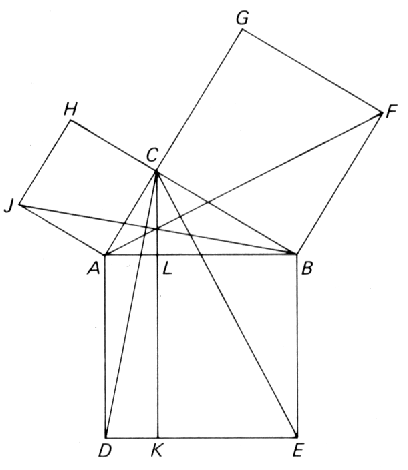7. One final example involving manipulatives and the Pythagorean Theorem is the following (from Eves).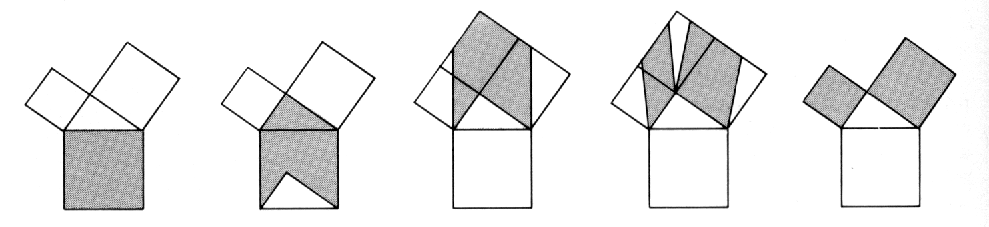8. The cardboard manipulatives can be misleading. Let's consider "The Enlarging Area Paradox" from section 2.2. A picture of the puzzle is given in problem 15 of section 2.2. It appears that an area of size 64 square units is manipulated into an area of size 65 square units.
9. Another example of such a manipulative trick is due to Paul Curry and can be found in Euclid's Window by Leonard Mlodinow (Touchstone, 2001).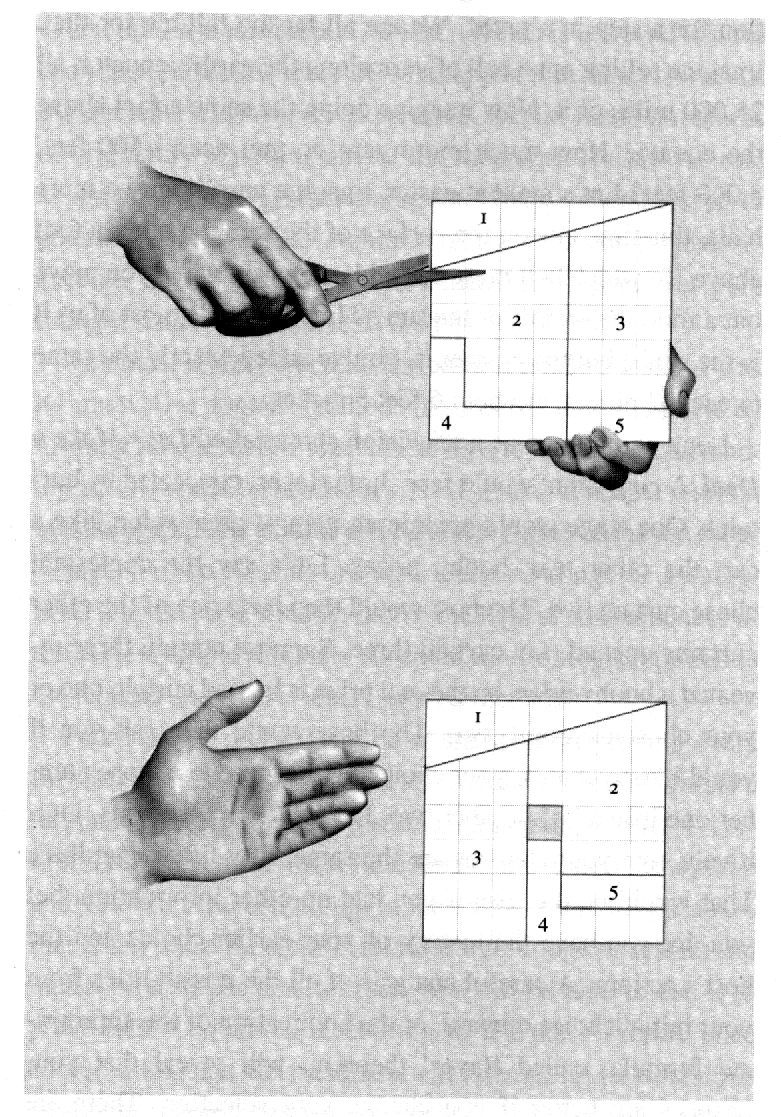Compass and Straightedge Constructions
(SOL: 3.19, 4.15, 6.16)

The Greeks were interested in drawing objects which could be constructed from nothing more than a compass (for drawing circles) and an unmarked straightedge (for drawing line segments). These restrictions allow them to transfer lengths of a given size, but not to measure lengths. The text has illustrations for bisecting an angle and constructing a perpendicular to a line. The text mentions that "handful of construction questions remained unanswered for more than 2000 years." These include (as stated by Eves): (1) The duplication of the cube, or the problem of constructing the edge of a cube having twice the volume of a given cube, (2) The trisection of an angle, or the problem of dividing a given arbitrary angle into three equal parts, (3) The quadrature of the circle, or the problem of constructing a square having an area equal to that of a given circle. These problems were solved in the 1800's using the area of math called abstract algebra. It was shown that each of these constructions is impossible with just a compass and straightedge.

The Parallel Postulate

The Parallel Postulate is what makes lines "straight" and planes "flat" (as we intuitively desire). The Parallel Postulate is neither true nor false - it is merely an assumption of Euclidean geometry. There are geometries in which the Parallel Postulate does not hold (i.e. noneuclidean geometries). In fact, there are places in our universe where the Parallel Postulate fails (since Einstein talks of curvature of spacetime). Even on the surface of the Earth, the Parallel Postulate has problems as we will see in section 4.6.

The Parallel Postulate has a long history, occasionally involving some controversy. It seems so self-evident that it should be "true" and should follow from more elementary principles. After 200 years of failure in proving the Parallel Postulate, attempts were made to find errors in assuming its negation. Instead of errors, noneuclidean geometry was discovered/invented. More of this in section 4.6. Some alternative (i.e. replacements) for the Parallel Postulate include (as stated in Eves):

1. If a straight line intersects two straight lines so as to make the interior angles on one side of it together less than two right angles, these straight lines will intersect, if indefinitely produced, on the side on which are the angles which are together less than two right angles (this is Euclid's famous 5th Postulate).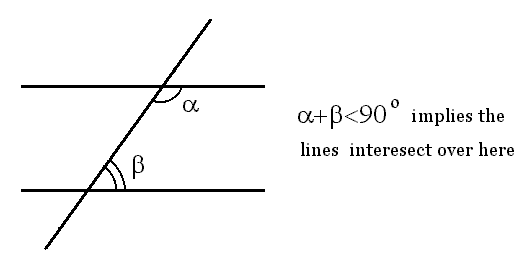2. There exists at least one triangle having the sum of its three angles equal to two right angles (i.e. equal in measure to 180o).
3. There exists a pair of similar non congruent triangles.
4. There exists a pair of straight lines everywhere equally distant from one another.
5. A circle can be passed through any three non collinear points.
6. Through any point within an angle less than 60o there can always be drawn a straight line intersecting both sides of the angle.

(SOL: 2.20)

< By repeatedly applying the Pythagorean Theorem, we can measure distances in 3-dimensions.

1. Suppose a room is 20 feet wide, 20 feet deep, and 8 feet high. How far apart are opposite corners of the room (such as the front left lower corner and the back right upper corner)?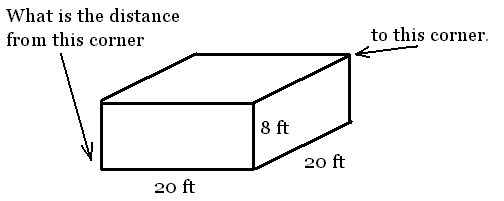2. A triangle is isosceles if two sides are of equal length. Find the length of the two unlabeled sides in the following isosceles triangle: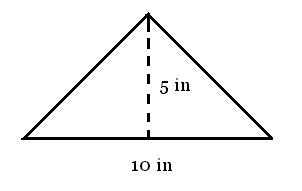3. The largest of the great pyramids in Egypt has base length 745 feet. What is the height of this pyramid? HINT: A cross section through the highest point and two opposite edges is an isosceles right triangle.

I Want a Widescreen TV!
(SOL:4.17, 7.11)

Standard televisions have a width to height ratio of 4:3. A widescreen TV has width to height ratio of 16:9. However, the sizes of TVs are always given in terms of a diagonal measure. This makes it quite tricky to compare the areas of TV screens, and VERY TRICKY to compare standard and widescreen TVs. Let's consider a number of questions related to dimensions and comparisons which use the Pythagorean Theorem.

1. I have a 27 inch (that is, diagonal measure of the screen) standard TV. What are the width and height of the screen? What is the area?
2. I want a 60 inch (diagonal measure) widescreen TV. What are the width and height of such a TV screen? What is the area?
3. When a widescreen image is displayed on a standard TV, black bars appear at the top and bottom of the screen. On my 27 inch TV, what is the diagonal measure of a widescreen image? HINT: You already know the width of the image from question 1.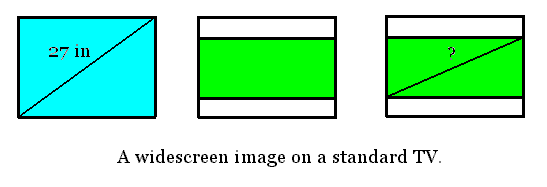4. When a standard image is displayed on a widescreen TV, black bars appear at the left and right of the screen. What is the diagonal measure of a standard image displayed on a 60 inch widescreen TV? HINT: You already know the height of the image from question 2.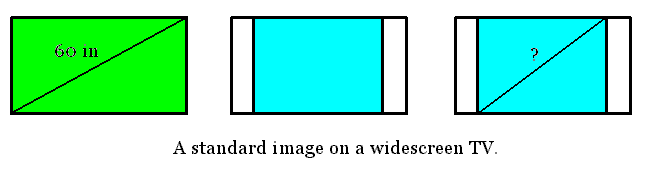5. My wife says we cannot afford a 60 inch widescreen TV (and... she's right... but I REALLY want one...). How large a widescreen TV would we have to get to still be able to display a 27 inch diagonal measure standard image?